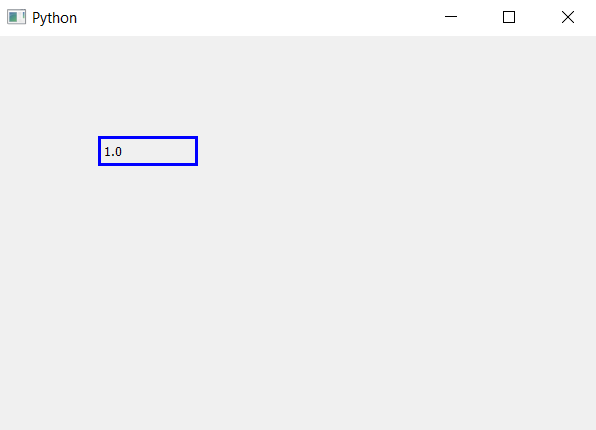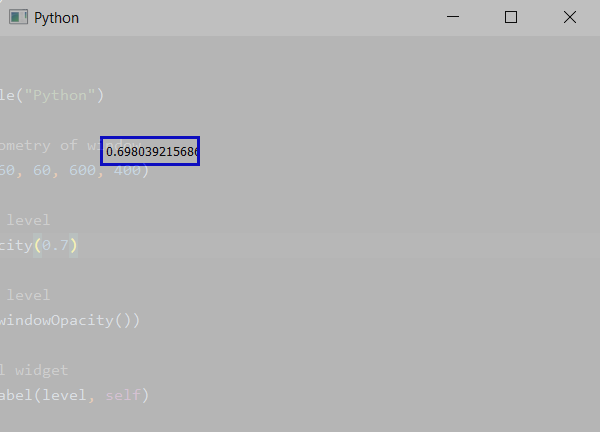Related Articles

# PyQt5 – Get opacity level of the main window | windowOpacity() method

• Last Updated : 26 Mar, 2020

`windowOpacity()` method allows us to see the opacity level of the main window. Opacity is the measure of impenetrability to electromagnetic or other kinds of radiation, especially visible light. In radiative transfer, it describes the absorption and scattering of radiation in a medium, such as a plasma, dielectric, shielding material, glass, etc.

Opacity level ranges from 0.0 to 1.0, where 0.0 means completely transparent and 1.0 means completely opaque, by default it is 1.0 i.e completely opaque.

Syntax : self.windowOpacity()

Argument : It takes no argument.

Return : Returns a float value ranging from 0.0 to 1.0

Example 1:

 `# importing the required libraries`` ` `from` `PyQt5.QtCore ``import` `*` `from` `PyQt5.QtGui ``import` `*` `from` `PyQt5.QtWidgets ``import` `*` `import` `sys`` ` ` ` `class` `Window(QMainWindow):`` ` ` ` `    ``def` `__init__(``self``):``        ``super``().__init__()`` ` ` ` `        ``# set the title``        ``self``.setWindowTitle(``"Python"``)`` ` `        ``# setting  the geometry of window``        ``self``.setGeometry(``60``, ``60``, ``600``, ``400``)`` ` `        ``# getting opacity level``        ``level ``=` `str``(``self``.windowOpacity())`` ` `        ``# creating a label widget``        ``self``.label_1 ``=` `QLabel(level, ``self``)`` ` `        ``# moving position``        ``self``.label_1.move(``100``, ``100``)`` ` `        ``# setting up the border``        ``self``.label_1.setStyleSheet(``"border :3px solid blue;"``)`` ` `        ``# show all the widgets``        ``self``.show()`` ` ` ` `# create pyqt5 app``App ``=` `QApplication(sys.argv)`` ` `# create the instance of our Window``window ``=` `Window()``# start the app``sys.exit(App.``exec``())`

Output :Example 2:

 `# importing the required libraries`` ` `from` `PyQt5.QtCore ``import` `*` `from` `PyQt5.QtGui ``import` `*` `from` `PyQt5.QtWidgets ``import` `*` `import` `sys`` ` ` ` `class` `Window(QMainWindow):`` ` ` ` `    ``def` `__init__(``self``):``        ``super``().__init__()`` ` ` ` `        ``# set the title``        ``self``.setWindowTitle(``"Python"``)`` ` `        ``# setting  the geometry of window``        ``self``.setGeometry(``60``, ``60``, ``600``, ``400``)`` ` `        ``# setting opacity level``        ``self``.setWindowOpacity(``0.7``)`` ` `        ``# getting opacity level``        ``level ``=` `str``(``self``.windowOpacity())`` ` `        ``# creating a label widget``        ``self``.label_1 ``=` `QLabel(level, ``self``)``        ``# moving position``        ``self``.label_1.move(``100``, ``100``)`` ` `        ``# setting up the border``        ``self``.label_1.setStyleSheet(``"border :3px solid blue;"``)`` ` `        ``# show all the widgets``        ``self``.show()`` ` ` ` `# create pyqt5 app``App ``=` `QApplication(sys.argv)`` ` `# create the instance of our Window``window ``=` `Window()``# start the app``sys.exit(App.``exec``())`

Output :Attention geek! Strengthen your foundations with the Python Programming Foundation Course and learn the basics.

To begin with, your interview preparations Enhance your Data Structures concepts with the Python DS Course. And to begin with your Machine Learning Journey, join the Machine Learning – Basic Level Course

My Personal Notes arrow_drop_up